# A step by step guide to the Counting Sort algorithmA detailed guide to the counting sort algorithm with implementations in C++, C# and Python.

While all comparison-based algorithms have a time complexity of O(nlogn) to sort an array of n elements, there are sorting algorithms running in linear time provided that some assumptions are verified.

Counting sort is one of such algorithms and it requires a precondition to be applied. Each of the n input elements shall be an integer in a finite range or shall have an integer key in that range.

Let's assume for now that the range is [0,k].

# The idea

The basic idea is that the number of times each key occurs in the input array (e.g. its frequency) can be used to determine the position of the elements in the sorted output array.

How is this possible?

Let's suppose that there is an input element with key equal to c, with c <= k. If we know that the input array contains m elements with key < c, the index of that element in the sorted output array will be clearly equal to m.

An example can help to understand better. If the input is [1,1,2,4,3,0,1,2,3,1], there are five keys less than 2. So the first 2 will go in position 5 in the output [0,1,1,1,1,2,2,3,3,4].

# Counting the frequencies

The frequency of each key can be computed using a bookkeeping array with size equal to k+1 initialized with zeros. Since the keys in the input array are in the range [0,k], we can iterate over it and use the keys as indices for the bookkeeping array.

During the iteration we increment the elements of the bookkeeping indexed by the keys. At the end, each element of the bookkeeping array will store the frequency of the key with the corresponding index.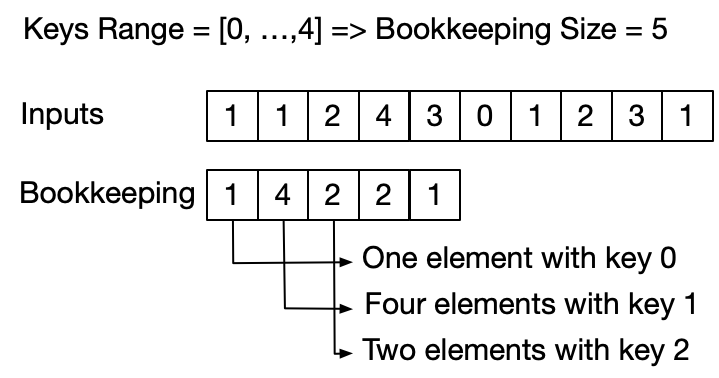# Building the sorted output

If there are only integer keys without attached values, it is trivial to build the sorted output. We can just iterate through the bookkeping array and fill the sorted output with the occurrences of each key.

void CountingSort(vector<int>& keys) {

int max_key = *max_element(keys.begin(), keys.end());

vector<int> bookkeeping(max_key + 1);

for (int key : keys) bookkeeping[key]++;

for (int i = 0, k = 0; i <= max_key; ++i) {
for (int j = 0; j < bookkeeping[i]; ++j) {
keys[k++] = i;
}
}
}


# Introduce the next_index array

The generic case where the input elements are key-value pairs is more complicated. It is necessary to precompute the position of each input element in the sorted output.

The easiest way is to accomplish this is building up another array. We can use the bookkeeping array to build up an array that will tell us where the next occurrence of a key goes in the sorted output.

We can call this array next_index because its i-th element represents the position of the next input element with key i in the sorted output. We can build the next_index array tasking into account two things:

1. the output position of the first element with key i corresponds to the number of elements with key < i

2. the bookkeeping subarray with indices in the range [0,i-1] contains the frequencies of all the elements with key < i

So the i-th element of the next_index array corresponds to the cumulative sum of the bookkeeping array up to the index i-1. Notice how the bookkeeping and the next_index arrays have the same size.

The last step is now using the next_index array to build the sorted output.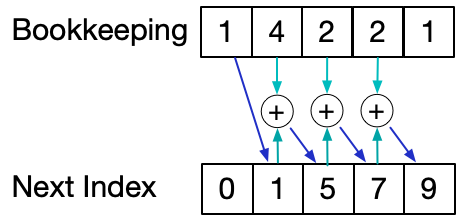# Building the sorted output using next_index

We can now iterate through the input array and use each key as an index for the next_index array to get its position in the output array.

Once the current element is placed in its output position, the next_index element indexed by its key get increased. This will always keep valid the invariant that the i-th element of next_index represents the position of the next input element with key i in the sorted output.

Once this iteration is completed, we can copy the sorted output array back to the input array.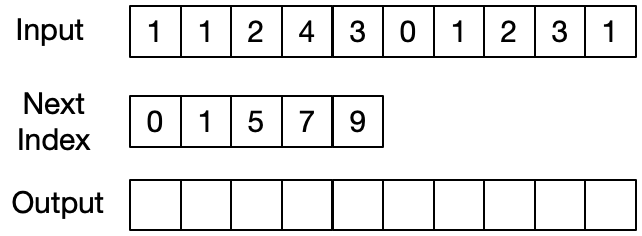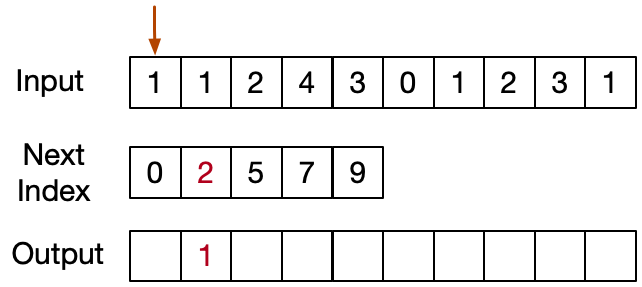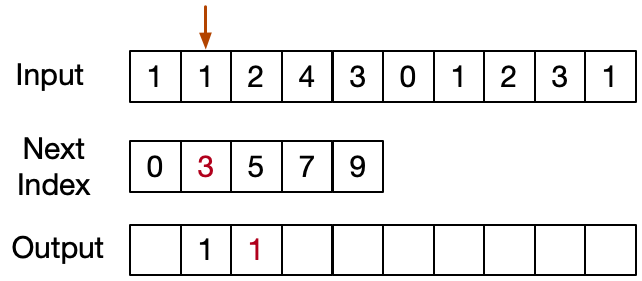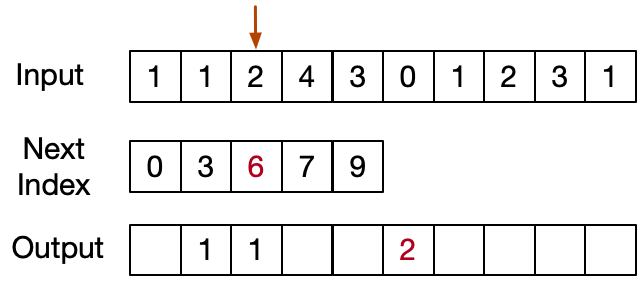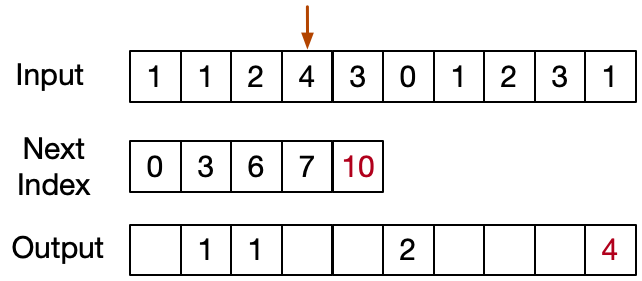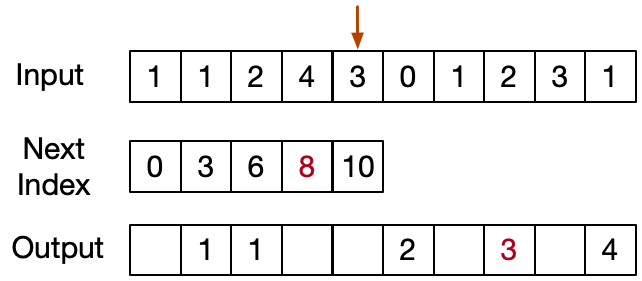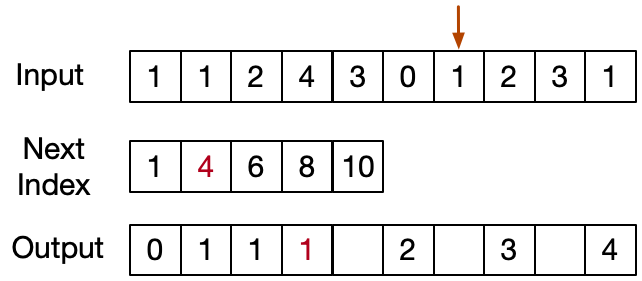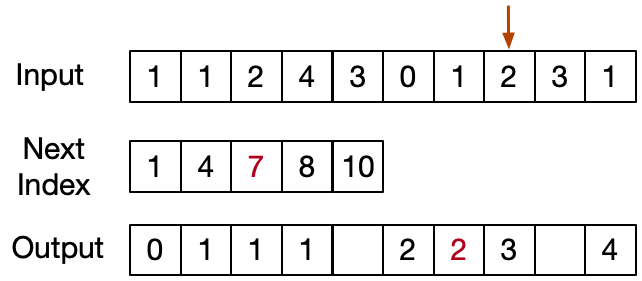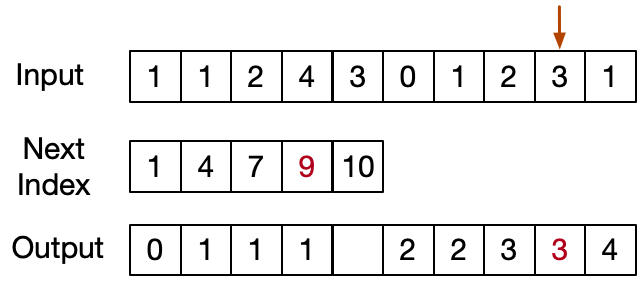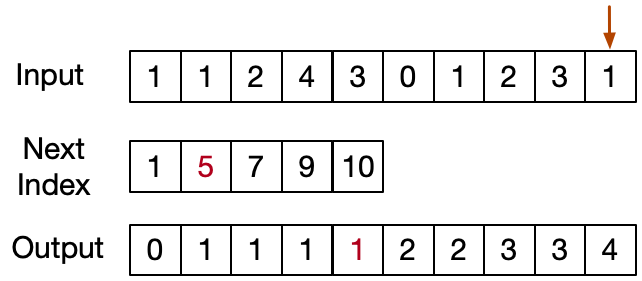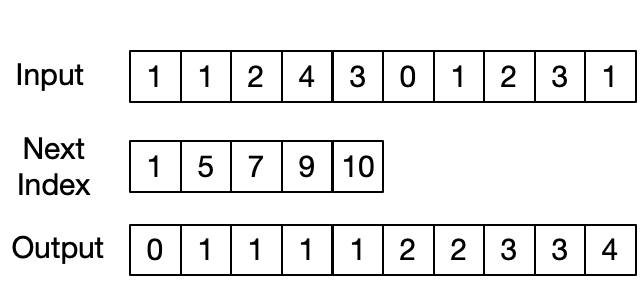vector<pair<int, string>> CountingSort(vector<pair<int, string>>& items) {

int max_key = max_element(items.begin(), items.end(),
[](auto const& x, auto const& y) {
return x.first < y.first;
})->first;

vector<int> bookkeeping(max_key+1, 0);

//counter[i] corresponds to the number of entries with key equal to i
for (const auto& item : items) {
bookkeeping[item.first]++;
}

//nextIndex[i] corresponds to the number of entries with key less than i
vector<int> next_index(max_key+1, 0);
for (int i = 1; i < next_index.size(); ++i) {
next_index[i] = next_index[i-1] + bookkeeping[i-1];
}

vector<pair<int, string>> output(items.size());
for (const auto& item : items) output[next_index[item.first]++] = item;

return output;
}


# Space optimization

Instead of creating a separate next_index array, we can use directly the bookkeeping array saving some memory space. To understand how, let's consider that:

1. the position of the last element with key i corresponds to the number of elements with key <= i

2️. the bookkeeping subarray with indices in the range [0,i] contains the frequencies of the elements with key <= i

So the cumulative sum of the bookkeeping array up to the index i corresponds to the position (plus 1) of the last input element with key i in the sorted output. The cumulative sum of the bookkeeping array can be computed in place.

We can then iterate backwards over the input array and use each key as an index for the bookkeeping array to get its position in the output array.

Before the current element is placed in its output position, the bookkeeping indexed by its key get decreased. This will always keep valid the invariant that the i-th element of next_index represents the position (plus 1) of the last input element with key i in the sorted output.

vector<pair<int, string>> CountingSort(vector<pair<int, string>>& items) {

int max_key = max_element(items.begin(), items.end(),
[](auto const& x, auto const& y) {
return x.first < y.first;
})->first;

vector<int> bookkeeping(max_key + 1, 0);

//count keys frequency
for (const auto& item : items) {
bookkeeping[item.first]++;
}

//at the end each element is the index of the last element with that key
std::partial_sum(bookkeeping.begin(), bookkeeping.end(), bookkeeping.begin());

vector<pair<int, string>> output(items.size());

//build sorted output iterating backward
for (auto it = items.crbegin(); it != items.crend(); ++it) {
output[--bookkeeping[it->first]] = *it;
}

return output;
}


# Extension to generic ranges

We always assumed that all the keys were positives and included in a range between 0 and some maximum value k.

Actually this is not a prerequisite. We can apply the counting sort to whatever integer range of keys. The trick is just to find the minimum element and store the frequency of that minimum element at 0 index.

# Properties

An important property of counting sort is that it is stable: elements with the same key appear in the output array in the same order as they do in the input array.

Regarding the asymptotic analysis we have instead that:

1. time complexity is O(n+k) to iterate through both the input array and the bookkeeping array

2. space complexity is O(k) to store the bookkeeping array.

Usually the number of items to be sorted is not asymptotically different than the number of keys those items can take on. In those cases k becomes O(n) and the time complexity of the whole algorithm is O(n). Anyway this is not always valid (i.e. input = [1e10,1,2,4,3,0,1,2,3,1]).

# Python implementation

def countingSort(input):

maxKey= max(input, key=lambda item:item)

bookeepingLength = maxKey+1
bookeeping =  * bookeepingLength

# Count keys frequency
for item in input:
bookeeping[item] += 1

# at the end each element is the index
# of the last element with that key
for i in range(1, bookeepingLength):
bookeeping[i] += bookeeping[i-1]

output =  * len(input)

# build sorted output iterating backward
i = len(input) - 1
while i >= 0:
item = input[i]
bookeeping[item] -= 1
position = bookeeping[item]
output[position] = item
i -= 1

return output


# C# implementation

class CountingSort {

private static int[] PartialSum(int[] input)
{
for (int i = 1; i < input.Count(); i++)
{
input[i] = input[i] + input[i - 1];
}

return input;
}

public static (int, string)[] CountingSort((int, string)[] items)
{
int max_key = items.Max(t => t.Item1);
var bookkeeping = new int[max_key + 1];

//count keys frequency
foreach (var item in items) {
bookkeeping[item.Item1]++;
}

//at the end each element is the index of the last element with that key
bookkeeping = PartialSum(bookkeeping);

var output = new (int, string)[items.Length];

//build sorted output iterating backward
for (int i = items.Length - 1; i >= 0; i--) {
output[--bookkeeping[items[i].Item1]] = items[i];
}

return output;
}
}


# Conclusion

Counting sort is a powerful and extremely efficient algorithm. Its basic idea is simple, but the implementation can be tricky and requires attention.

This post provided a step by step guide for implementing the counting sort algorithm.

The implementation of the algorithm in multiple programming languages (C++, C# and Python) is available at my GitHub repository.

If you liked this post, follow me on Twitter to get more related content daily!